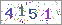Letters: A B C D E F G H I J K L M N O P Q R S T U V W X Y Z

The Logarithmic Potential: Discontinuous Dirichlet and Neumann Problems
 Date: 21 April 2011, 11:18Free register and download UseNet downloader, then you can FREE Download from UseNet.     Download without Limit " The Logarithmic Potential: Discontinuous Dirichlet and Neumann Problems " from UseNet for FREE! This book studies fundamental properties of the logarithmic potential and their connections to the theory of Fourier series, to potential theory, and to function theory. The material centers around a study of Poisson's integral in two dimensions and of the corresponding Stieltjes integral. The results are then extended to the integrals in terms of Green's functions for general regions. There are some thirty exercises scattered throughout the text. These are designed in part to familiarize the reader with the concepts introduced, and in part to complement the theory. The reader should know something of potential theory, functions of a complex variable, and Lebesgue integrals. The book is based on lectures given by the author in 1924-1925 at the Rice Institute and at the University of Chicago.TABLE OF CONTENTS I. PRELIMINARY CONCEPTS. STIELTJES INTEGRALS AND FOURIER SERIES1. Functions of limited variation. 2. Continuation of the preceding. 3. Integrals with respect to a function of limited variation.4. Note on the second law of the mean. 5. Classical theorems on integrals and limits of integrals. 6. The limits of Stieltjes integrals.7. Note on Lebesgue integrals. 8. Convergence of Fourier series. 9. Summability of series.II. FUNCTIONS HARMONIC WITHIN A CIRCLE 10. Preliminary theorems. 11. A preliminary result. 12. Note on integral identities. 13. Digression: Functions of points and of point sets.14. Properties of the Poisson-Stieltjes integral. 15. Continuation of the preceding: Behaviour of u in the neighborhood of the boundary.16. The Poisson integral: F absolutely continuous.III. NECESSARY AND SUFFICIENT CONDITIONS. THE DIRICHLET PROBLEMS FOR THE CIRCLE17. Fundamental theorem and lemma. 18. Proof of fundamental theorem. 19. Special cases of the Poisson integral.20. The Dirichlet problem and its extension.IV. POTENTIALS OF A SINGLE LAYER AND THE NEUMANN PROBLEM21. The Stieltjes integral for potentials of a single layer. 22. Necessary and sufficient conditions. 23. Further properties.24. The Neumann problems. 25. General points of view. 26. Digression: Physical interpretation of a general distribution of mass.27. Cauchy's integral formula.V. GENERAL SIMPLY CONNECTED PLANE REGIONS AND THE ORDER OF THEIR BOUNDARY POINTS28. Conformal transformations and general regions. 29. Invariant forms of conditions (i), (ii) etc. 30. Invariant forms of conclusions.31. Order of boundary points. 32. Integrals on the boundary and the Dirichlet problems.33. Special cases of the condition (ii). The continuous boundary value problem.34. A new continuous boundary value problem. 35. The generalized Neumann problem in the general region.VI. PLANE REGIONS OF FINITE CONNECTIVITY36. Functions harmonic outside a circle. 37. The multiply connected region bounded by n+1 distinct circles.38. Representation in terms of the Green's function. 39. Boundary integrals and Stieltjes integral equations.40. General regions of finite connectivity. Isolated point boundaries. 41. Annular regions. Determination of the functions F and F.42. Uniqueness of the representation of Theorem 3 for S.VII. RELATED PROBLEMS43. A simple discontinuous boundary value problem. 44. Continuous boundary value problems. 45. Regions with continuous boundaries.46. Regions with rectifiable boundaries. 47. Regions of infinite connectivity. 48. Remarks on necessary and sufficient conditions.49. Convergence in the mean of positive order less than one. 50. Integro-differential equations of Bocher type.

### DISCLAIMER:

This site does not store The Logarithmic Potential: Discontinuous Dirichlet and Neumann Problems on its server. We only index and link to The Logarithmic Potential: Discontinuous Dirichlet and Neumann Problems provided by other sites. Please contact the content providers to delete The Logarithmic Potential: Discontinuous Dirichlet and Neumann Problems if any and email us, we'll remove relevant links or contents immediately.###Comments (0) All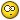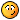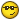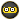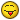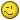Verify: